List of parts

Electronic Explorer Board ($330 /$660)
Nexys 3 Board ($190 /$270)
54 Capacitors (C2C DAC)
R,C circuit
Breadboard PCB like Electronic Explorer (EBay)

VHDL Files for Nexys 3 Board for Spartan 6, XC6SLX16, Package CSG324
Programming Bit File for Nexys 3 Board for Spartan 6, XC6SLX16, Package CSG324

6 Laboratories

Description

The system uses a NEXYS3 FPGA board for Sigma Delta converter clock generation and filtering the output data stream, the Electronic Explorer to generate an input signal and measure signals with the oscilloscope and a DAC to generate waveforms controlled by the FPGA.
A FPGA is used to be able to accurately control the high speed timing behaviour.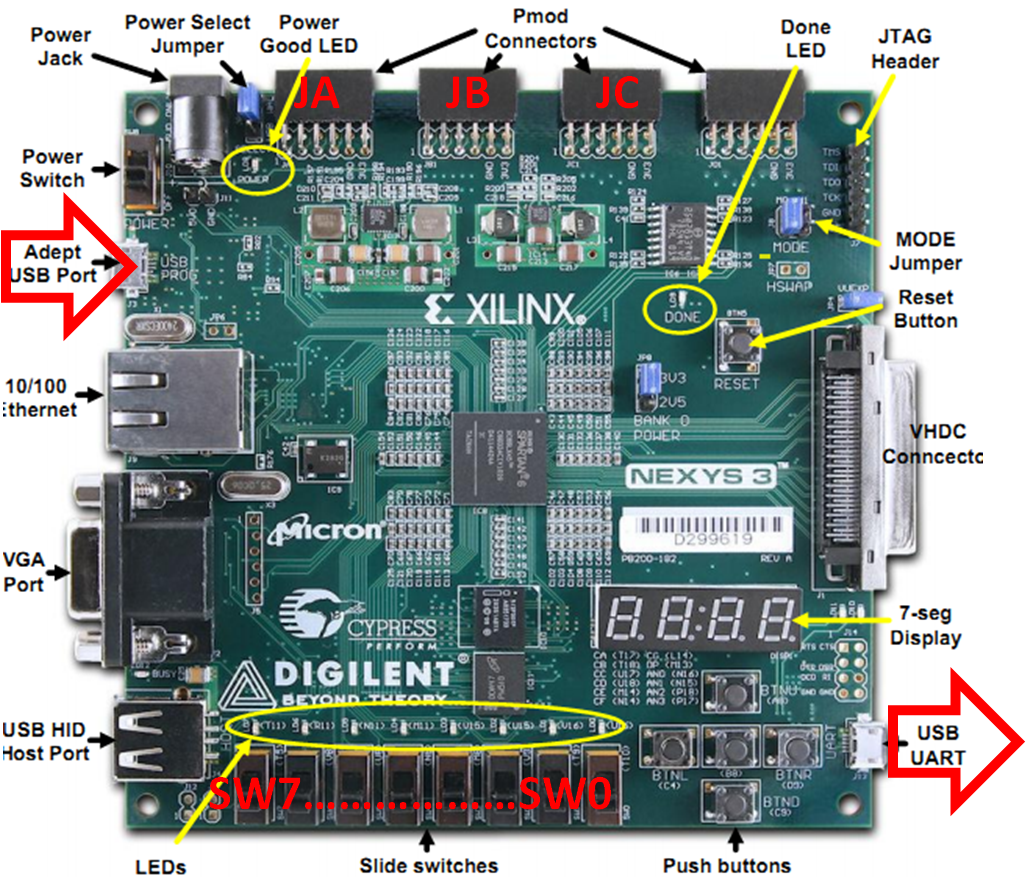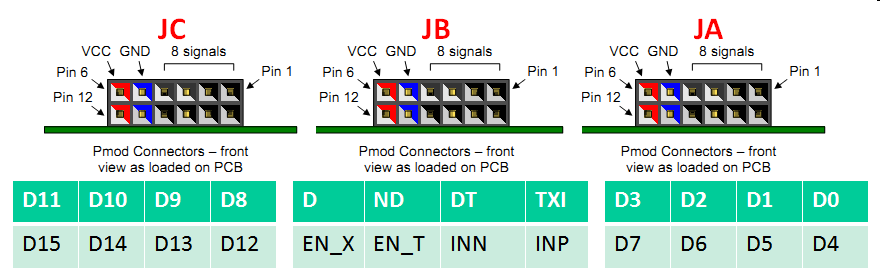INN and INP are the inputs of the comparator of the sigma delta converter.
D and ND are the normal and inverted outputs of the comparator.
DT toggles each time a new value is generated from the SINC filter.
TXI is the transmit line of serial connection.

Operation of the Nexys 3 FPGA board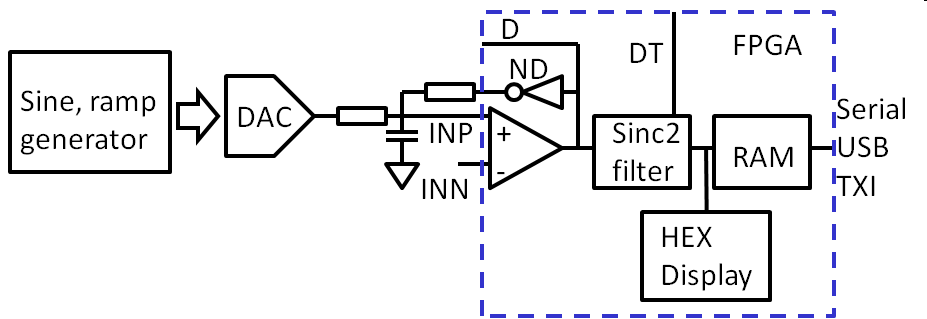Figure: Block diagram of the setup.

The Nexys3 board has a comparator with differential inputs (INP, INN) as 1-bit ADC (IBUFDS_inst).
The output is fed to a SINC2 filter (SINC2) with 26 Bits.
DT toggles each time a new value is generated from the SINC filter.
The sample rate and decimation can be controlled with sw(2..0).
Since the serial interface has only 38400 Baud and the conversion can be faster, a dual port RAM buffer (Inst_RAM) is implemented with a size of 2WBUFFER = 16k, 16-bit output values taken from the upper Bits of the SINC2 filter output.
A UART is used to stream a serial output of data to the 2nd USB port.
The serial interface streams a new line for each output data word in hexadecimal.
Example:
x01E80
G007B1
x007B2

The buffer is read continously and a start of the buffer is marked with an G instead of an x.

Connect the ground from the FPGA, DAC and EE board.

Since the configuration of the FPGA board is not preconfigured, it can be loaded with the Adept tool, through the USB port at the upper left side.
Start Adept, select tab Config, 'Browse' for a bit file
and then 'Program' it.
The switches on the lower left control the mode of operation:

 switch (2 downto 0) D length tCLKD DT length tSample oversampling fbw ENOB 000 40 ns 80 us 2048 6.25 kHz 001 40 ns 160 us 4096 3.125 kHz 010 150 ns 160 us 1024 3.125 kHz 011 150 ns 650 us 4096 0.75 kHz 100 630 ns 650 us 1024 0.75 kHz 101 630 ns 10 ms 16k 50 Hz 110 10 us 10 ms 1024 50 Hz 111 2.5 us 10 ms 4096 50 Hz
• switch (4 downto 3) control operation mode
• 00 SAR operation
• 01 sawtooth down DAC (16 Bit)
• 10 sawtooth up DAC (16 Bit)
• 11 sine signal DAC (43 periods, 8 bit, 512 length)
• switch (6 downto 5) control number of periods of sine signal
• 00 1: period
• 01 9: periods
• 10 5: periods
• 11 43: periods
• switch (sw7) = 1 controls enabling inverted data for capacitive C2C.
The 7 segment display shows the result of the ADC sigma delta conversion.

Connect AWG2 (compare level) of the Electronic Explorer to INN and set it to a DC voltage of 1.75 V.
Connect AWG1 (input signal) of the Electronic Explorer to INP and set it to a DC voltage of 1.75 V. With the oscilloscope of the Electronic Explorer measure AWG1, DT and TXI.

Make a typical screenshot.

Confirm the length of DT, the oversampling, bandwidth and expected ENOB for the switch positions of sw(2..0) on the board.
Make a table to calculate minimum input signal frequency and test duration for a ramp and sine test signal for the switch positions and available DRAM buffer.

Attach the DAC from the previous laboratory to pins D15..D6 and measure the ramp signal and sine signal (switch 6..3).
A USB cable can be attached to the serial USB port (red arrow, lower right FPGA board). putty (Hyperterminal) can be started with 38400 baud rate, 8 Databits, no parity and 1 stopbit, no flow control on the PC and connected to a COM port to transfer data from the FPGA board to the PC. Sometimes the connection has to be tried twice.

Connecting the RC network and starting measurements

Calculate values for the RC network. Take into account the series resistance of your DAC.

Connect the RC network of the passive first order sigma delta modulator to JB pin ND. The INP of the JB connector of the FPGA port is the signal input for the ADC and is connected to the capacitance C. The AWG1 of the Electronic Explorer is connected as signal generator via R1 to the C of the sigma delta modulator.
The INN of the JB connector is connected to AWG2 providing the compare voltage 1.75 V.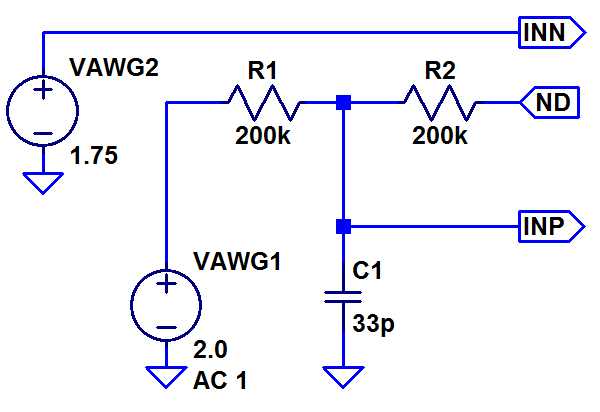Check set up:

Change the voltage level on AWG1 and observe the change on the 7 segment display.
Attach putty (Hyperterminal) and establish a data connection. There is an option to save the received data to a file. Look at the file in Excel or Matlab.

Measure INL and DNL of the sigma delta modulator

Do 4 measurements applying an external and internal ramp and sine signal and log the data via putty (Hyperterminal). Calculate and INL, DNL.

Measure SNR of the sigma delta modulator

Generate a sine wave with AWG1 and DAC and record the output data with putty (Hyperterminal) and do a FFT to determine signal to noise ratio and ENOB.

Final Questions

What are the performance limits of the investigated sigma delta modulator? (Resolution, sample rate) How can the performance be improved?
Do you have a proposal for a 2nd order passive sigma delta modulator?

Image of measurement setup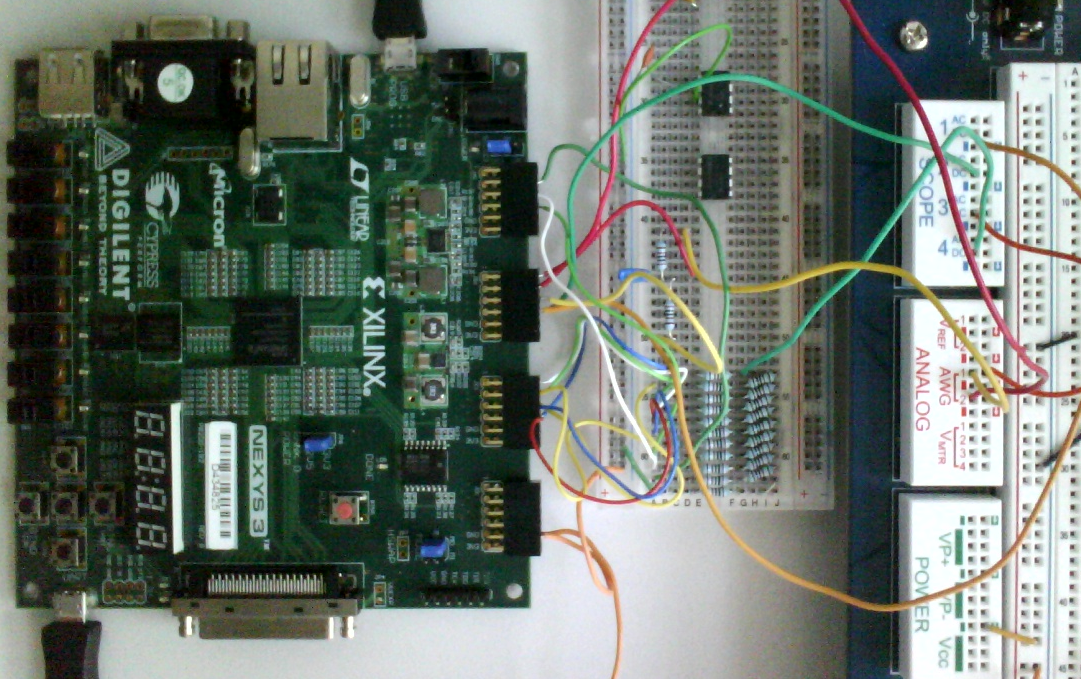Figure: Physical test setup with 10 bit R2R DAC

Solution

DAC Sine signal source quality

How is the sine generated?
What resolution does the sine DAC have?

switch(2..0) = 000; tCLKD = 40 ns; fCLKD = 25 MHz

Effective number of bits ENOB:

$SNR = 6.02 \cdot N_{Bit} + 1.76 - 5.17 + 30 log OSR$

An oversampling of 2048 should give an increase in SNR of $30 log OSR \approx 95 dB$.
Since the sigma delta modulator has a 1 bit output NBit = 1.
ENOB:
$ENOB = \frac{95 - 5.17 +1.76 }{6.02} \approx 15$

Bandwidth:
OSR · tCLKD = 2048 · 40 ns = 80 μ s gives one data point.
For fbw 2 points are needed.
$f_{bw} = \frac{1}{2 \cdot 80 \mu s} = 6.25kHz$

Minimum input signal frequency:
One period in 16k points.
$f_{sig,min} = \frac{1}{16 \cdot 1024 \cdot 80 \mu s} = 0.763 Hz$
Time for getting 16k data, one periodof sine signal:
$T = \frac{1}{f_{sig,min}} = 1.31 s$

Measurement of the sine signal period for sw(6,5)=00; sw(4,3)=11.
16k points sine lookup table or oscilloscope measurement confirms 16k points.
10 Bit DAC means 10..16 points per code.
For FFT use 43 periods to get peak at higher frequency.

RC dimension:
$\delta t = 40 ns$
12 Bits to have lowest fgRC = 64 fbw = 400 kHz
$R C = \frac{1}{2 \cdot \pi \cdot 400 kHz} = 397 ns$
Sample and Hold C requirement for 12 Bit, 3.3V -> C > 80 fF
For example: R = 10 k Ω C = 10 pF
R C = 100 ns

Data processing

Identify and copy a 16k block in the log file.
Transfer hex values to decimal values.
For a ramp or sawtooth signal identify one period and extract the values for a histogramm evaluation.
For a sine signal confirm a prime integer number of cycles or apply a windowing function for FFT.
Do the FFT and calculate signal to noise values.

The ADC data received by the serial interface of the PC is analysed using FFT Javascript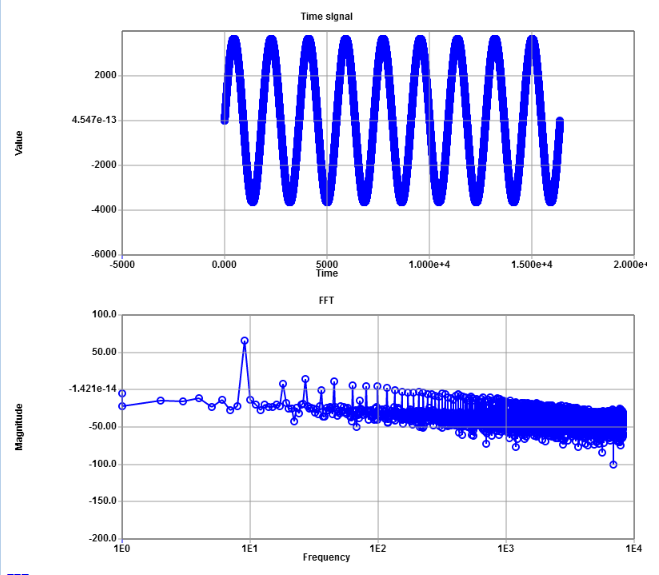Signal level is 65.19 dB and the total noise 18.07 dB.
This gives SNR = 47.12 dB, which is ENOB = SNR / 6.07 dB = 7. 76 Bits.

The distortion with harmonics can be seen in the spectrum, coming from non linearities.
Not taking into account distortions a total noise magnitude of 8.82 dB can be seen.
This gives SNR = 56.63 dB, which is ENOB = SNR / 6.07 dB = 9. 28 Bits.

Unfortunately quality of sine signal is not given.
Electronic Explorer has a 10-bit DAC. R2R DAC with 0.1% resistance can also give 10-bit.
Probably signal to noise is limited by the quality of the DAC.

Diagnosis of a sigma delta ADC

If the theoretical SNR is not reached a diagnosis is needed.

Clock jitter

Clock jitter will show up using test signals of various frequencies.
With clock jitter the noise will increase with higher frequency test signals, since dV/dt will be bigger and consequently the error will be bigger at higher frequencies.

Inter symbol interference

Inter symbol interference changing the digital output waveform depending on the output code pattern can be studied with varying sample rates. Lower sample rates and clock frequencies should give less inter symbol interference noise.

Signal and power supply noise

Signal and power supply noise is caused by external signal sources and can show up as harmonics or random noise. Shielding and buffer capacitances can be used to lower ths noise. With active sigma delta modulators the input range can be increased with higher power supply voltages. If the SNR is increasing with high power supply voltages shielding und buffer capacitances are not sufficient.

Varying oversampling rate

Higher oversampling rate should give more bits ENOB and SNR.

Summary

16 bit digital code output for sawtooth, sine

 Clock frequency, sampling 25 MHz Sanpling period 40 ns OSR 2048 Bandwidth 6.25 kHz Voltage range Vref 3.3 V DAC Bits 10 DAC Expexted SNR 60 dB Expected SNR (oversampling) 95 dB Expected ENOB (oversampling) 15 Measured SNR 47 dB Measured ENOB 7.76 Measured SNR No harmonics 56 dB Measured ENOB 9.28 Measured LSB 15.2 mV

Outlook

• Update the FPGA board
• Update sine signal generation to more, 16 Bits
R2R or discrete DAC with serial interface
• Audio application 44kHz, 24 Bits